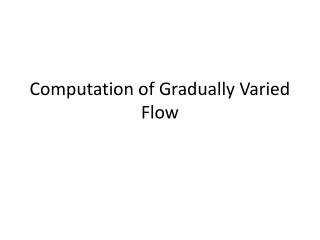DownloadDownload Presentation# Computation of Gradually Varied Flow

Télécharger la présentation## Computation of Gradually Varied Flow

- - - - - - - - - - - - - - - - - - - - - - - - - - - E N D - - - - - - - - - - - - - - - - - - - - - - - - - - -
##### Presentation Transcript

1. Computation of Gradually Varied Flow

2. Introduction • We have covered sketching water surface profiles in channel qualitatively. • Water surface profile calculations determine the water surface elevations along the channel length for a specified discharge. • It is necessary to compute the flow conditions in these flows for engineering applications that include planning, design and operation of open channels to assess their effects.

3. Remarks • We will use the continuity, momentum, and energy equations that relate various flow variables such as flow depth, discharge, flow velocity at different sections of a channel to determine the flow conditions • Known inputs are: • Channel cross section • Manning, n • Channel bottom slope • Rate of discharge • At different channel sections solve for: • Flow depth • Flow velocity

4. Remarks • Continuity Equation: • Energy Equation: (Choudhry, 2008)

5. Remarks • Gradually Varied flow profile: , = distance along the channel (measured positive in the downstream direction), longitudinal slope of the channel bottom, slope of the energy grade line, top water surface width, and acceleration due to gravity. • First order ordinary differential equation in which is the independent variable and is the dependent variable. • This equation describes the rate of variation of flow depth with respect to distance . • The rate of variation of flow depth is function of the properties of the channel section, flow depth, and discharge. • Several procedures to compute water surface profiles have been developed and implemented in various engineering software programs (HEC-RAS)

6. Direct Step Method • The statement of our problem is: The flow depth at distance is known; determine distance where a specified flow depth will occur. The properties of the channel section, , and are known. • The slope of the energy grade line will be referred to as friction slope is a function of (flow depth, , varies with distance, ) • Average friction slope • Geometric mean friction slope • Harmonic mean friction slope • Laurenson  suggested that (Choudhry, 2008)

7. Direct Step Method • We can therefore say that: = By knowing the location of section 2 this becomes the starting value for the next step. Then, by successively increasing or decreasing the flow depth and determining where these depths will occur, the water surface profile may be computed.

8. Cons • The flow depth is not computed at the predetermined locations. Interpolations may become necessary if the flow depths are required at specified locations. Similarly, the cross sectional information has to be estimated if such information is available only at the given locations. • Cumbersome to apply to non-prismatic channels.

9. Example • A trapezoidal channel having , , , and carries a discharge of . Compute the backwater profile created by a dam which backs up the water to a depth of immediately behind the dam. The upstream end of the profile is assumed at a depth equal to greater that the normal depth. The energy coefficient